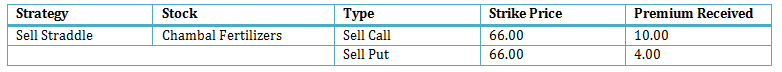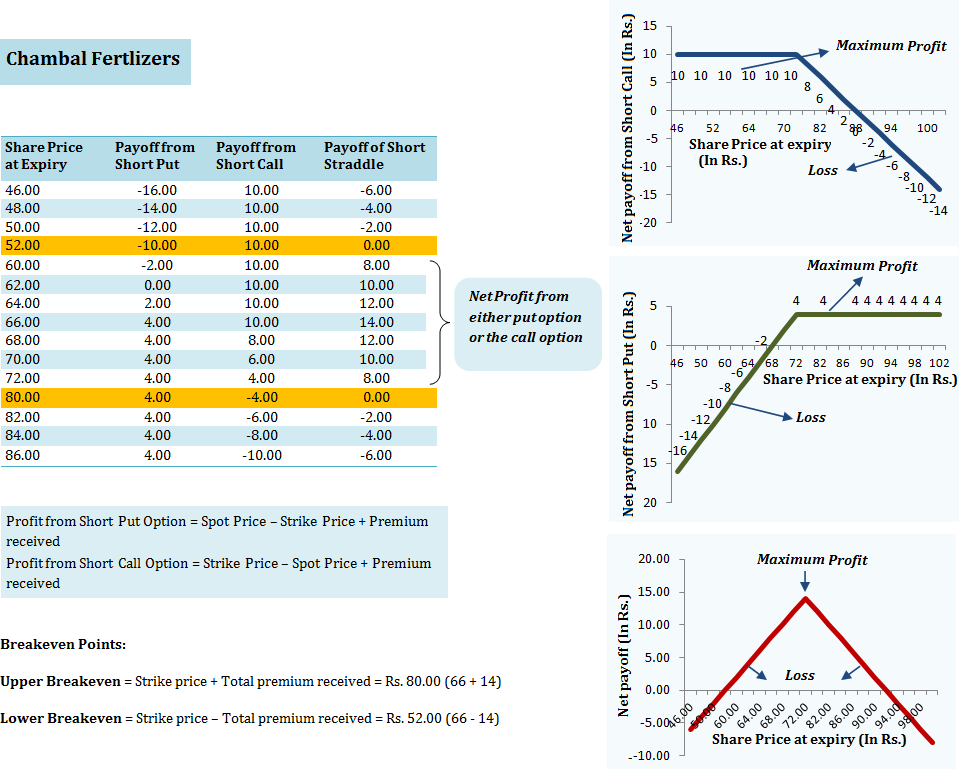When to use: Short straddle option strategy is used when the investor believes that the stock is not very volatile. The idea is to earn an option premium on two option contracts. The investor believes that the stock price will not change much before the expiry date. The maximum profit is the amount of premium collected by writing the options.

How it works: In short straddle option strategy, you sell both a call option and a put option for the same stock, each with the same strike price, and the same expiration date, T.

For example: On 16th August 2013, you write a call option at a premium of Rs. 10 and a put option at a premium of Rs. 4 of Chambal Fertilizers, expiring 26th September 2013 with a Strike Price of Rs. 66. The share was trading at Rs. 63 on that day. The total initial gain is the sum of both premiums, which is Rs. 14.Risk/Reward: In short straddle option, the risk involved is unlimited and the reward/profit is limited to the option premium received on both – the call option and the put option.

For Sell Call – If the price of Chambal Fertilizers stays below Rs. 66.00 (i.e. the strike price), until expiry, you will retain the entire premium amount on the call option (i.e. Rs. 10.00). If however the price rises above Rs. 66.00, the buyer of call option may exercise his option and make a profit based on how far above Rs. 66.00 does the stock price rise. From the perspective of the seller of the call (you), you will start suffering a loss once the stock price rises above Rs. 76.00 (i.e. the strike price + premium).

For Sell Put – If the price of the share stays below Rs. 66.00 (i.e. the strike price) until expiry, you will retain the entire premium amount (i.e. Rs. 4.00). If however the price falls below Rs. 66.00, the buyer of put option may exercise his option and make a profit based on how far below Rs. 66.00 does the stock price fall. From the perspective of the seller of the put (you), you will start suffering a loss once the stock price falls below Rs. 62.00 (i.e. the strike price – premium).

In order for this trade to realise profit at expiration the stock must be below Rs. 80.00 or above Rs. 52.00. The table below show the net payoff of short straddle strategy at different spot prices on expiry:

(click to enlarge)The table above allows you to easily see the break-even points, maximum profit and the loss potential at expiry in rupee terms.

The two break-even points occur when the price of the underlying share equals Rs. 52.00 and Rs. 80.00.

The maximum loss will be incured if the share price upon expiry is below Rs. 52.00 or above Rs. 80.00 and the maximum profit which the investor can make is equal to the total premium received on both the options i.e. Rs. 14.00.

## How to use Short Straddle Option Strategy Excel calculator

Just enter your expected spot price on expiry, option strike price and the amount of premium, to estimate your net pay-off from the Short Straddle Option Strategy.

Note: The example and calculations are based assuming a single share though in reality options are based on lots of many shares. For example Chambal Fertilizers option contract is for 4000 shares. Accordingly the premium you receive will be Rs. 56,000 for 2 lots (i.e. 10.00*4000 + 4.00*4000) in our example.

Also Note: Unlike the buyer of an option who only pays the premium to buy the option, the seller of an option must deposit a margin amount with the exchange. This is because he practically takes an unlimited risk as the stock price may fall to any level (up to zero). In case the price falls sharply below the strike price, the exchange utilises the margin amount to make good the profit which the option buyer makes. The amount of margin is decided by the exchange and it typically ranges from 15 % 60 % based on the volatility in the underlying stock and market conditions. In the above example, as a seller of both put option and call option, you will have to deposit a margin of Rs. 90,393.60 (i.e. Strike price * Lot size * 17.12%) for selling/writing a lot of Chambal Fertilizers’ put option and call option. Note that the total value of your outstanding position in this case will be Rs. 5,28,000 (i.e. strike price * lot size)